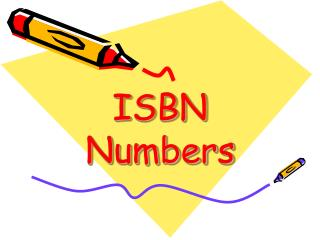DownloadDownload PresentationISBN Numbers

# ISBN Numbers

Download Presentation## ISBN Numbers

- - - - - - - - - - - - - - - - - - - - - - - - - - - E N D - - - - - - - - - - - - - - - - - - - - - - - - - - -
##### Presentation Transcript

1. ISBN Numbers

2. Every book published has an ISBN number • ISBN is the International Standard Book Number • The last digit or character of the number is called the check digit

3. A check digit means that a computer can check if the code for the book is valid • How does the computer check?

4. Answer ………. • By using multiplication tables and then multiples of 11

5. Write down the first 25 multiples of 11

6. Do you notice a pattern?

7. 11 22 33 44 55 66 77 88 99 110 121 132 143 154 165 176 187 198 209 220 231 242 253 264 275

8. What is the 34th multiple of 11?

9. 34 x 11 • Open out the 34 3…. 4 • Add the 3+4, insert the 7 in the middle • 374 • 34 x 11 = 374 • Is it magic? • Why does this work? Does it always work?

10. How about 45 x 11 ? • 4……… 5 • 4+5 = 9 • 495 • So 45 x 11 = 495

11. How about 65 x 11 ? • 6……..5 • 6 + 5 = 11 • 6115 • But 65 x 11 is not 6115 • 65 x 11 = 715 • What happens here? 6… 5 1 1 ____ 715

12. Why does this work? • 27 x 11 is 10 x 27 = 270 + 1 x 27 = 27 11 x 27 = 297 Look what happens to the digits in the sum

13. Calculate……… • 72 x 11 • 48 x 11 • 61 x 11

14. What should be added to 456 so that it can be divided by 11 with no remainder? • What should be added to 285 to make it a multiple of 11?

15. Back to ISBN numbers • What does ISBN stand for? • What is the last digit or character called? • What is this last check digit for? • How does the computer do this?????

16. The book “Year 5 Solving Problems” has the ISBN number 0 7136 5448 1 • The last digit is the check digit, 1

17. 0 7136 5448 1 • How the computer works out the 1…. 0 x 10 = 0 7 x 9 = 63 1 x 8 = 8 3 x 7 = 21 6 x 6 = 36 5 x 5 = 25 4 x 4 = 16 4 x 3 = 12 8 x 2 = 16 • Total 197 • What should be added to 197 to make it a multiple of 11? • Next multiple of 11 is 198, so add 1. This is the check digit.

18. “Red Herrings and White Elephants”ISBN number 1 84358 129 #? • Find the check digit #

19. 1 8 4 3 5 8 1 2 9

20. 1 x 10 = 8 x 9 = 4 x 8 3 x 5 x 8 x 1 2 9

21. 1 x 10 = 10 8 x 9 = 72 4 x 8 = 32 3 x 7 = 21 5 x 6 = 30 8 x 5 = 40 1 x 4 = 4 2 x 3 = 6 9 x 2 = 18 Total 233 Next multiple of 11? 242 Check digit must be 9

22. Think about the possible check digits, what could they be? • 0, 1, 2, 3, 4, 5, 6, 7, 8, 9 or 10 • There is only one space for the check digit, so the 10 becomes Roman, X

23. Find some books for yourself and calculate the check digit